# The figure shows angular position versus time graphs for six different objects. a) Rank these graphs on the basis of...

The figure shows angular position versus time graphs for six different objects.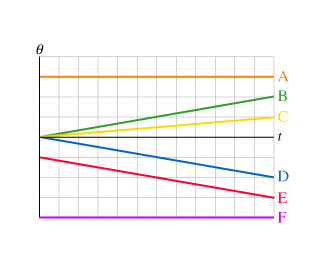a) Rank these graphs on the basis of the angular velocity of each object. Rank positive angular velocities as larger than negative angular velocities. Rank from largest to smallest. To rank items as equivalent, overlap them.

b) Rank these graphs on the basis of the angular acceleration of the object. Rank positive angular accelerations as larger than negative angular accelerations.Rank from largest to smallest. To rank items as equivalent, overlap them.

Concepts and reason

The required concepts to solve these questions are angular velocity and angular acceleration.

Initially, find the slope of each of the line and then calculate the angular velocity for every point and rank from largest to smallest. Similarly, calculate the angular acceleration and then rank from largest to smallest.

Fundamentals

Angular velocity is defined as the rate of change of the angular position with respect to time.

The expression for angular velocity is,

$\omega = \frac{{\Delta \theta }}{{\Delta t}}$

Here, $\theta$ is angular position and $t$ is time.

Angular acceleration is defined as the rate of change of the angular velocity with respect to time.

The expression for angular acceleration is,

$\alpha = \frac{{\Delta \omega }}{{\Delta t}}$

Here, $\omega$ is angular velocity and is $t$ the time.

The expression for slope is,

${\rm{Slope}} = \;\frac{{{\rm{change in quantity along }}y{\rm{ - axis}}}}{{{\rm{change in quantity along }}x{\rm{ - axis}}}}$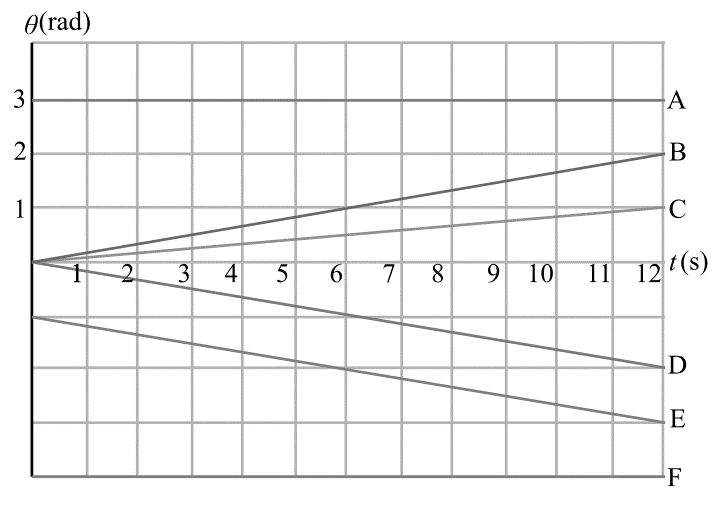(a)

The expression for slope of the line (angular velocity) is,

${\rm{Slope}} = \;\frac{{\Delta \theta }}{{\Delta t}}$

The slope of the line A and F is zero because velocity is constant.

The slope of the line B is,

${\rm{Slope}} = \;\frac{{{\theta _1} - \theta }}{{{t_1} - t}}$

Substitute $2{\rm{ rad}}$ for ${\theta _1}$ , $0{\rm{ rad}}$ for $\theta$ , $12{\rm{ s}}$ for ${t_1}$ and $0{\rm{ s}}$ for $t$ in above equation.

$\begin{array}{c}\\{\rm{Slope}} = \;\frac{{2{\rm{ rad}} - 0{\rm{ rad}}}}{{12{\rm{ s}} - 0{\rm{ s}}}}\\\\ = \frac{1}{6}{\rm{rad}} \cdot {\rm{s}}\\\end{array}$

The slope of the line C is,

${\rm{Slope}} = \;\frac{{{\theta _2} - \theta }}{{{t_2} - t}}$

Substitute $1{\rm{ rad}}$ for ${\theta _2}$ , $0{\rm{ rad}}$ for $\theta$ , $12{\rm{ s}}$ for ${t_2}$ and $0{\rm{ s}}$ for $t$ in above equation.

$\begin{array}{c}\\{\rm{Slope}} = \;\frac{{1{\rm{ rad}} - 0{\rm{ rad}}}}{{12{\rm{ s}} - 0{\rm{ s}}}}\\\\ = \frac{1}{{12}}{\rm{rad}} \cdot {\rm{s}}\\\end{array}$

Slope of the line D is,

${\rm{Slope}} = \;\frac{{{\theta _3} - \theta }}{{{t_3} - t}}$

Substitute $- 2{\rm{ rad}}$ for ${\theta _3}$ , $0{\rm{ rad}}$ for $\theta$ , $12{\rm{ s}}$ for ${t_3}$ and $0{\rm{ s}}$ for $t$ in above equation.

$\begin{array}{c}\\{\rm{Slope}} = \;\frac{{ - 2{\rm{ rad}} - 0{\rm{ rad}}}}{{12{\rm{ s}} - 0{\rm{ s}}}}\\\\ = - \frac{1}{6}{\rm{rad}} \cdot {\rm{s}}\\\end{array}$

Slope of the line E is,

${\rm{Slope}} = \;\frac{{{\theta _4} - \theta }}{{{t_4} - t}}$

Substitute $- 3{\rm{ rad}}$ for ${\theta _4}$ , $- 1\;{\rm{rad}}$ for $\theta$ , $12{\rm{ s}}$ for ${t_3}$ and $0{\rm{ s}}$ for $t$ in above equation.

$\begin{array}{c}\\{\rm{Slope}} = \;\frac{{ - 3{\rm{ rad}} - \left( { - 1} \right){\rm{ rad}}}}{{12{\rm{ s}} - 0{\rm{ s}}}}\\\\ = - \frac{1}{6}{\rm{rad}} \cdot {\rm{s}}\\\end{array}$

Rank of angular velocities from largest to smallest is,

${\rm{B}} > {\rm{C}} > {\rm{A}} = {\rm{F}} > {\rm{D = E}}$

(b)

The expression for angular acceleration is,

$\alpha = \;\frac{{d\omega }}{{dt}}$

In the graph, they all have constant slopes. It means that all lines represent constant angular velocity. Thus,

$\begin{array}{c}\\\alpha = \;\frac{{d\left( {{\rm{constant}}} \right)}}{{dt}}\\\\ = 0\\\end{array}$

Rank of angular acceleration from largest to smallest is ${\rm{D = E}} = {\rm{B}} = {\rm{C}} = {\rm{A}} = {\rm{F}}$ .

Ans: Part a

Rank of angular velocities from largest to smallest is ${\rm{B}} > {\rm{C}} > {\rm{A}} = {\rm{F}} > {\rm{D = E}}$ .

b,c (a,f) (D,E)

source: mastering physics

Here ,

angular velocity is given as

angular velocity , w = d(theta)/dt

d(theta)/dt is the slope of theta vs Time graph

from the graph ,

the order of slope of lines from largest to lowest is

1. B

2. C

3. A = F

5. D = E

Rank these graphs on the basis of the angular acceleration of the object. Rank positive accelerations as larger than negative angular acceleration

#### Earn Coin

Coins can be redeemed for fabulous gifts.

Similar Homework Help Questions
• ### Rank the six combinations of electric charges on the basis of the electric force acting on...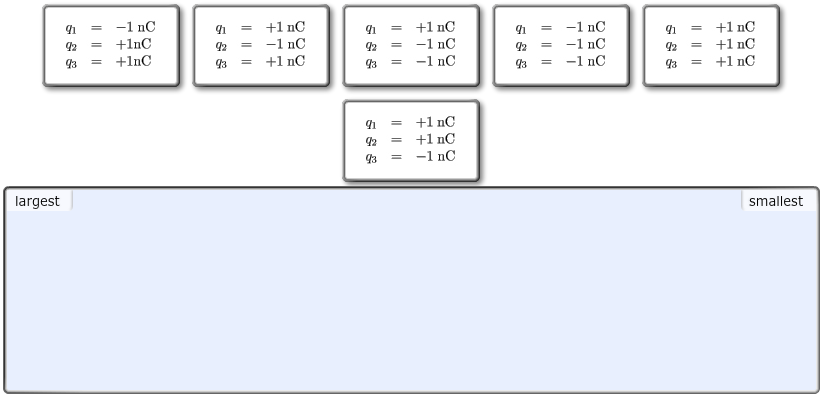Rank the six combinations of electric charges on the basis of the electric force acting on q1. Define forces pointing to the right as positive and forces pointing to the left as negative. Rank positive forces as larger than negative forces.  Rank from largest to smallest, placing the largest on the left and the smallest on the right. To rank items as equivalent, overlap them.  The correct ranking cannot be determined.

• ### Rank the six combinations of electric charges on the basis of the electric force acting on...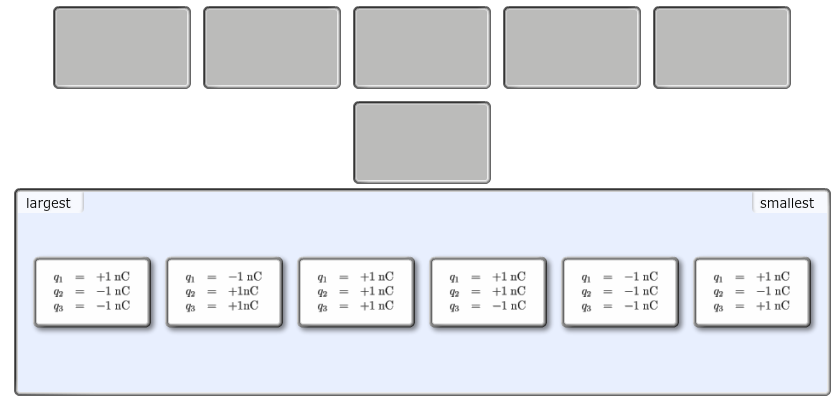Rank the six combinations of electric charges on the basis of the electric force acting on q1. Define forces pointing to the right as positive and forces pointing to the left as negative. Rank positive forces as larger than negative forces.  Rank from largest to smallest, placing the largest on the left and the smallest on the right. To rank items as equivalent, overlap them.  There are two types of charge, which have opposite signs. Which type we call "positive" is...

• ### Part A Rank in order, from largest to smallest, the angular accelerations α a   to α...

Part A Rank in order, from largest to smallest, the angular accelerations α a   to α d   in the figure(Figure 1) . Rank from largest to smallest. To rank items as equivalent, overlap them.

• ### The six metals have the work functions, W. Part A Rank these metals on the basis...

The six metals have the work functions, W.Part A Rank these metals on the basis of their cutoff frequency. Rank from largest to smallest. To rank items as equivalent, overlap them.Part B Rank these metals on the basis of the maximum wavelength of light needed to free electrons from their surface. Rank from largest to smallest. To rank items as equivalent, overlap them.Part C Each metal is illuminated with 400 nm (3.10 eV) light. Rank the metals on the basis of the maximum...

• ### Take a look at the six position vs time graphs below. Rank these graphs, considering the...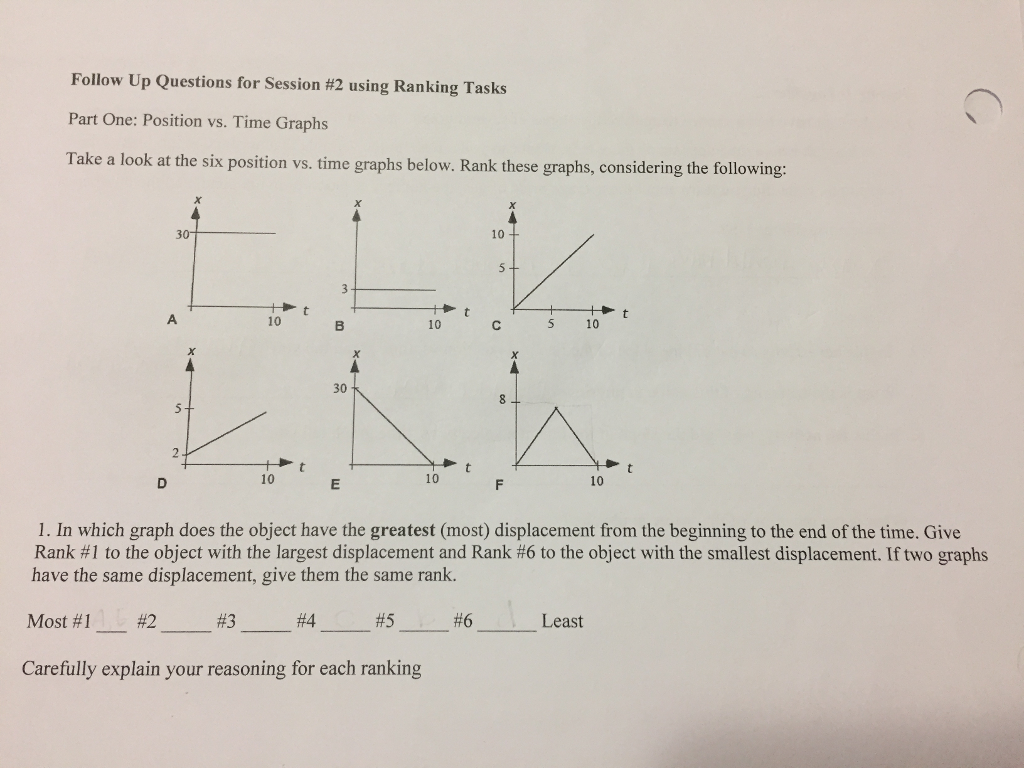Take a look at the six position vs time graphs below. Rank these graphs, considering the following:​ Follow Up Questions for Session #2 using Ranking Tasks Part One: Position vs. Time Graphs Take a look at the six position vs. time graphs below. Rank these graphs, considering the following: 10 + Ht A 10 10 t 30 D 10 10 1. In which graph does the object have the greatest (most) displacement from the beginning to the end of the...

• ### parts A,B&C please! Conceptual Exercise 13.12 A position-versus-time plot for an object undergoing simple harmonic motion...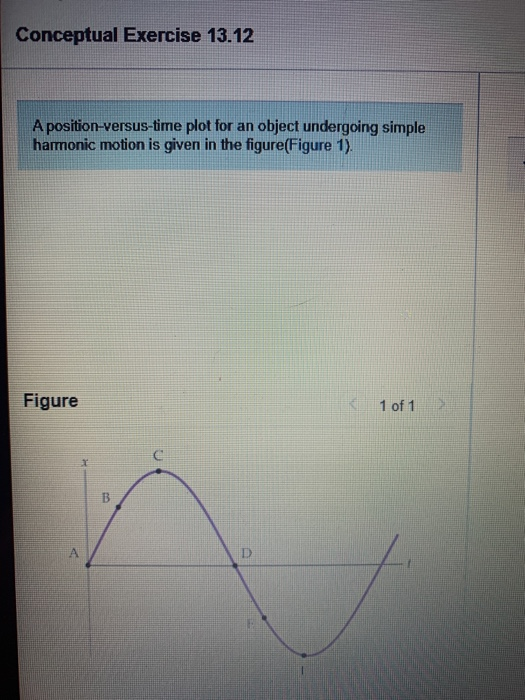parts A,B&C please! Conceptual Exercise 13.12 A position-versus-time plot for an object undergoing simple harmonic motion is given in the figure(Figure 1). Figure 1 of 17 Part A Rank the six points indicated in the figure in order of decreasing speed. Rank points from highest to lowest speed. To rank items as equivalent, overlap them. Reset Help O O O OOO Highest Lowest The corTec Part B Rank the six points indicated in the figure in order of decreasing velocity....

• ### Rank each pendulum on the basis of the maximum kinetic energy it attains after release. Rank...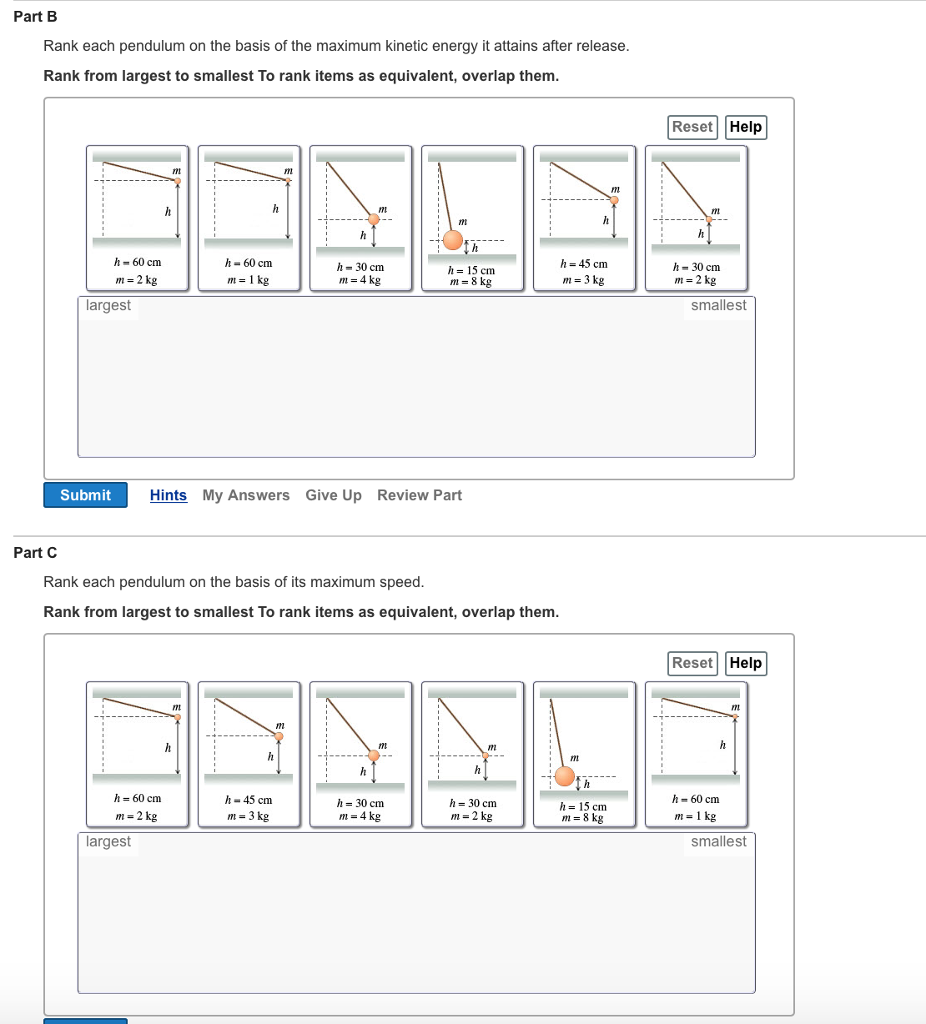Rank each pendulum on the basis of the maximum kinetic energy it attains after release. Rank from largest to smallest To rank items as equivalent, overlap them. Rank each pendulum on the basis of its maximum speed. Rank from largest to smallest To rank items as equivalent, overlap them.

• ### There were 2 parts. I was confused how to rank from large to small.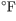Six objects are placed in a 500 (260) oven and allowed to reachthermal equilibrium. Each object has a mass of 1.0 .The specific heat and thermal conductivity of each substance are denoted by and Part B:When removing the objects from the oven, you accidentally touch each one with your hand. Rank these objects on the basis of how hot they feel.Rank from largest to smallest. To rank items as equivalent, overlap them.http://i43.tinypic.com/148ow3a.jpgPart C:Each of the objects is immediately dunked in...The figure shows angular position versustime graphs for six different objects.Rank these graphson the basis of the angular velocity of each object. Rank positiveangular velocities as larger than negativeangularvelocities.Rank fromlargest to smallest. I think thevelocities are ranked as such: b=d=e>c>a=fCan someoneplease check me?Thank you!!!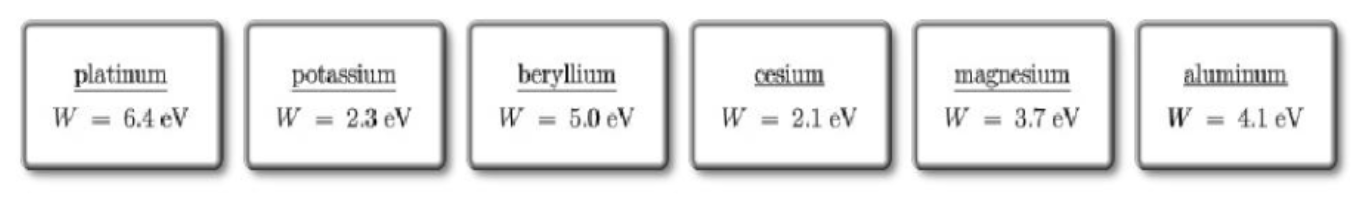The six metals have the work functions W. Part ARank these metals on the basis of their cutoff frequency. Rank from largest to smallest. To rank items as equivalent, overlap them.  Part BRank these metals on the basis of the maximum wavelength of light needed to free electrons from their surface. Rank from largest to smallest. To rank items as equivalent, overlap them.  Part CEach metal is illuminated with 400 nm (3.10 eV) light. Rank the metals on the basis of the maximum...